## Multiphase Flow Modeling : Part 2 - Terminologies

The terminologies used in the study of multiphase flows are as follows :

Continuous phase or Primary phase : This is basically the carrier fluid, that is present in bulk.

Dispersed phase or Secondary phaseThis is the discrete phase which is usually in the form of  particles, droplets, or bubbles.

The secondary phase may or may not have a distinct velocity field i.e. it will at some times follow the continuous phase or at times will have its own velocity field.Volume fraction :

Volume fraction of a dispersed phase : It is defined as the ratio of volume of dispersed phase to the volume of the medium or the total volume. Mathematically represented by :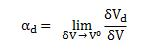Where del Vd is the volume of the dispersed phase in  volume del V. Unlike a continuum, the volume fraction cannot be defined at a point and is defined based on the volume.

Volume fraction of a continuous phase : Similarly, defined as the ratio of volume of continuous phase to the volume of the medium or the total volume. Mathematically represented by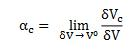where del Vc is the volume of the continuous phase in the volume. By definition the sum of the volume fraction must be unity i.e.Densities :

In multiphase we have two terms for densities, the bulk density and the material density. The bulk density is defined as the mass of the dispersed phase per unit volume of mixture, mathematically given by,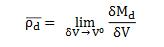del Md mass of dispersed phase. This bulk density is related to the material density rho(d) by,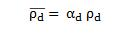The sum of the bulk densities for the dispersed and continuous phases is the mixture density.

Velocities :

There are generally two terms used to define velocities, the superficial velocity and the phase velocity.

Superficial Velocities : It is mostly associated with pipe flow and is the mass flow rate, of the respective phase divided by the pipe area A and material density. So in case of dispersed phase this superficial velocity is given by: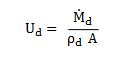Phase velocities : The phase velocity Ud is the actual velocity of the phase. The volume fraction relates the phase velocity with the superficial velocity as follows :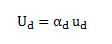The phase velocity or the bulk density, are generally defined for our convenience purpose in study of multiphase flow and different from the actual velocity and density of the phase. They are obtained by multiplying actual velocity or density with volume fraction.

Mass concentration of dispersed phase :

It is the ratio of the mass of dispersed phase to that of the continuous phase in a mixture.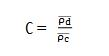Quality :

It is the measure of the quantity of dispersed phase in the medium (both the dispersed and continuous phase).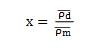It is the ratio of mass flux of the dispersed phase to that of continuous phase.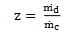Disperse Flows :

In such flows the phase loading of dispersed flows is comparatively lower (by mass) than the continuous phase. The bulk of the flow is occupied by the continuous phase and dominates the flow phenomena. In dispersed flow the particle motion is mainly controlled by the fluid forces (like drag and lift).

Dense Flows :

Such flows are generally associated with high phase loadings of dispersed phase. Here the interparticle collisions play a dominant role in the flow field controlling the overall particle motion.

The dense flows are classified into two main categories namely :

Collision dominated : Collisions between the particles control the overall flow features.
Contact dominated : Continuous particle to particle contact controls the overall flow features.

Stokes Number :

It is a non-dimensional number, given by the ratio of  particle (dispersed phase) relaxation time to the characteristic time scale of the flow. Mathematically represented by: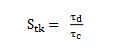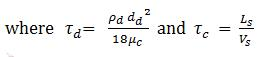Significance of Stokes Number :

If Stk << 1 , then particles follow the continuous phase flow closely.
If Stk > 1 , then dispersed phase particles will move independent of the continuous phase flow.

{module }